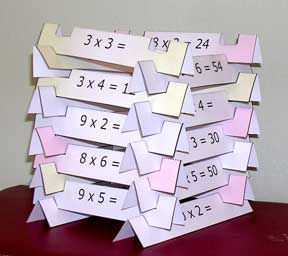# Archivo del sitio

## Tablas del 6, 7, 8 y 9 en sus manos!

At the age of 8 I had to learn the multiplying tables. I’ve never been good at memorizing lists or tables. It was easy to learn the tables from 1 to 5 but from 6 to 9 it seemed to be way more complicated… A year later I heard this trick on the radio and it saved my life. Since then I’ve taught it to many other kids. I passed such a bad time at school as I was the only one in my class who didn’t know the tables so I hope this trick was useful for any parent or teacher who knew any child in this situation

## Step 1Ascribe values

– First put your hands in front of you as shown in the drawing
– In each hand, ascribe a value from 6 to 10 to each finger

## Step 2How to multiply

Step 1

Choose the numbers to multiply. Example: 7×8

Step 2

Put together the fingers whoses values you want to multiply.

Step 3

Now count the touching fingers and the ones below them. The number you get will be the tens. Example: 5

Step 4

Now multiply the fingers above the ones touching of the left hand and the ones in the right hand. The number you get will be the units. Example: 3×2=6

**In some cases you will get a number of units bigger than nine, in that case sum both quantities**

Example: 7×6

– Touching fingers + the ones below  ->  3

– Fingers above the ones touching in left hand  ->  3
3 x 4 = 12
– Fingers above the ones touching in the right hand  ->  4

3        (tens)
Now we’ve got 3 tens and 12 units  ->                  + 12      (units)
———
42     (final result)

## Step 3Another trick for the table of 9

Here’s an extra trick for the whole table of nine.

– First put your hands in front of you
– Then ascribe values from 1 to 10 to your fingers
– Fold the finger whose value you want to multiply nine times
– The fingers remaining unfolded in the left will be the tens
– The fingers remaining unfolded in the right will be the units

Example:  9 x 4

– Fold the fourth finger
– Fingers remaining unfold in the left  –3  (tens)
– Fingers remaining unfold in the right  ->  6 (units)
– Final result  ->  36

Anuncio publicitario

## Aprender las tablas de multiplicar – Multiplication Thinkin’ LogsMultiplication Thinkin’ Logs

Blogged with the Flock Browser